## group of students to run 100 m.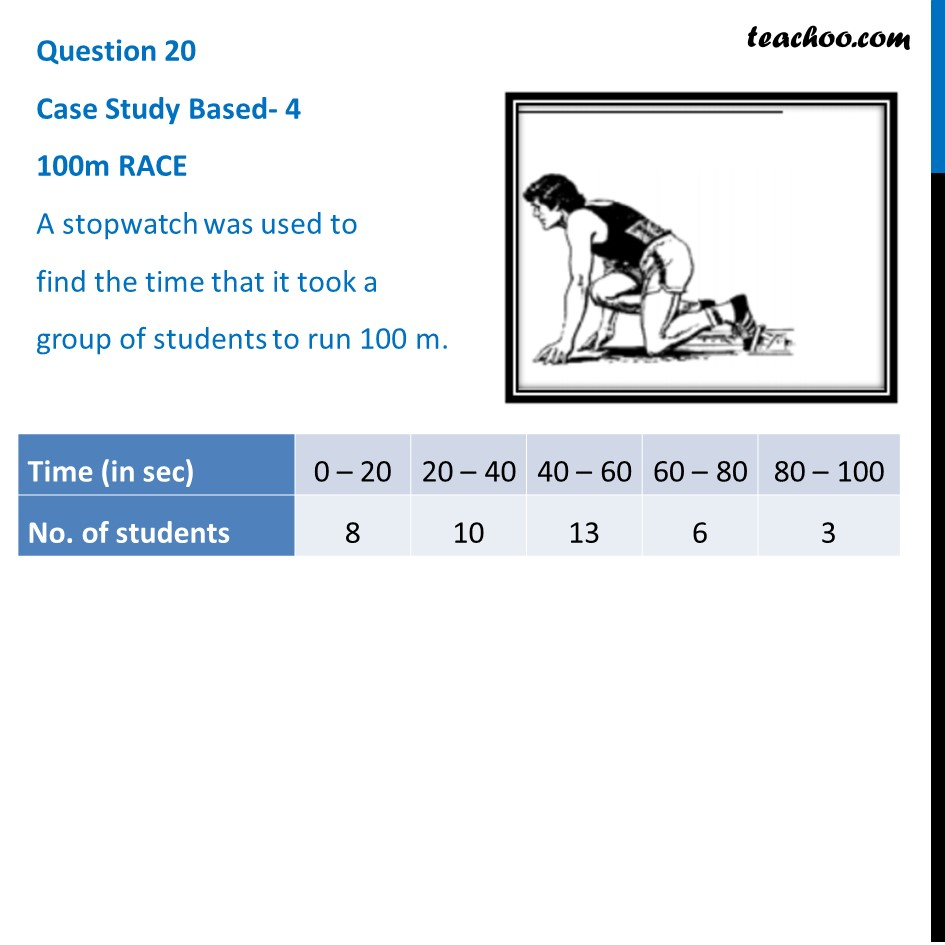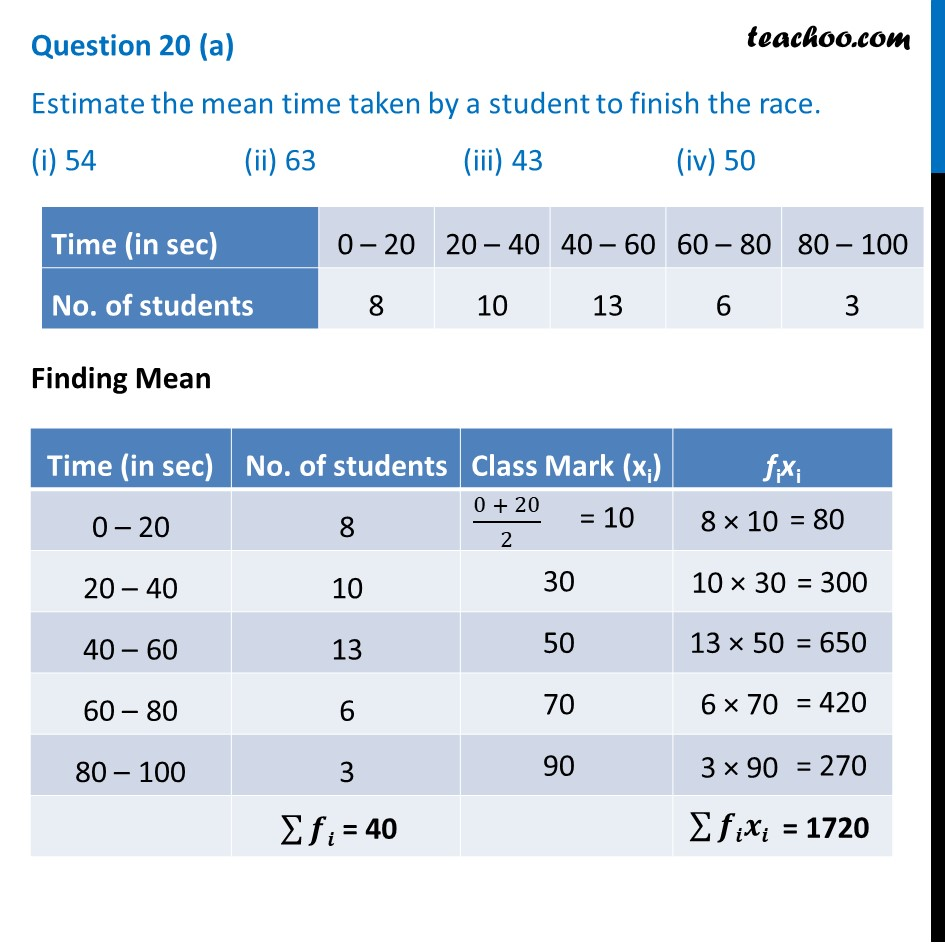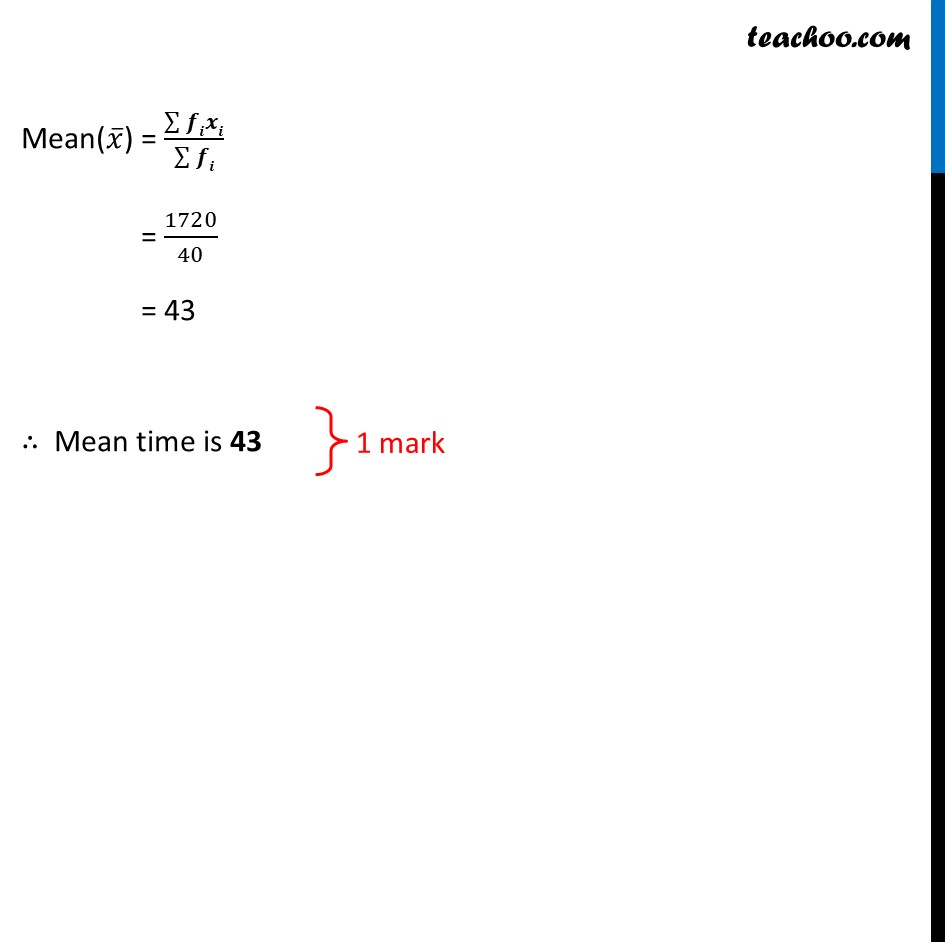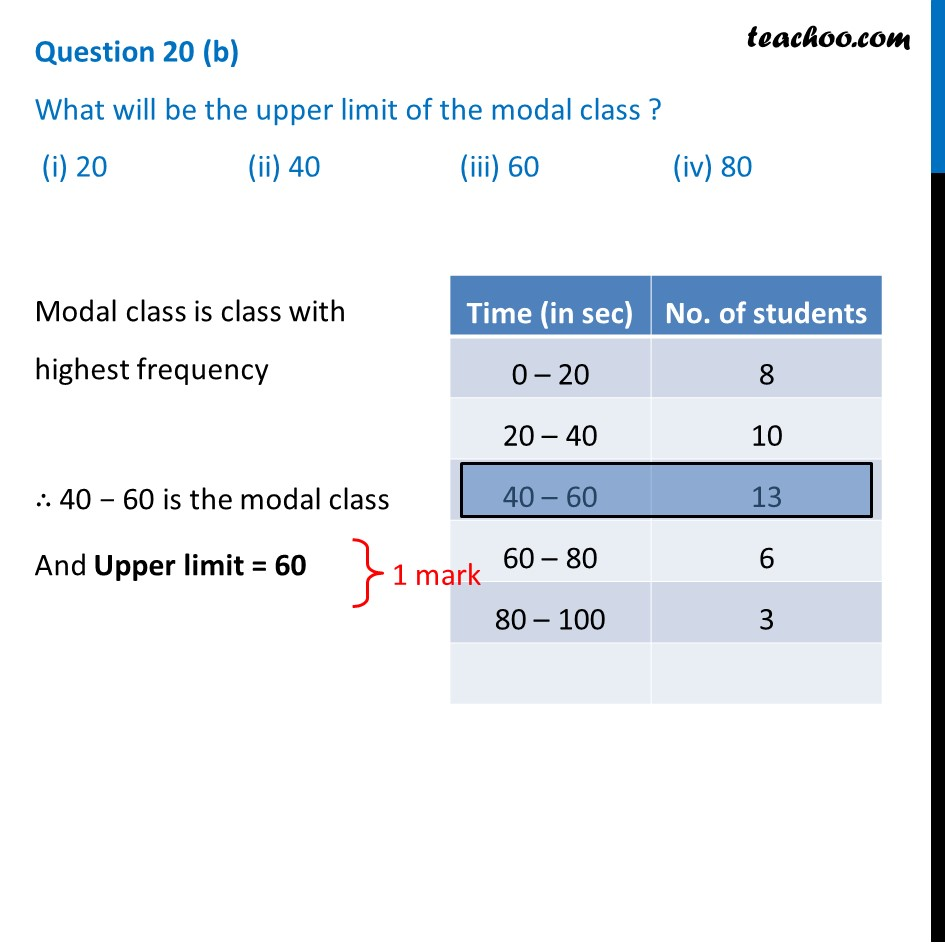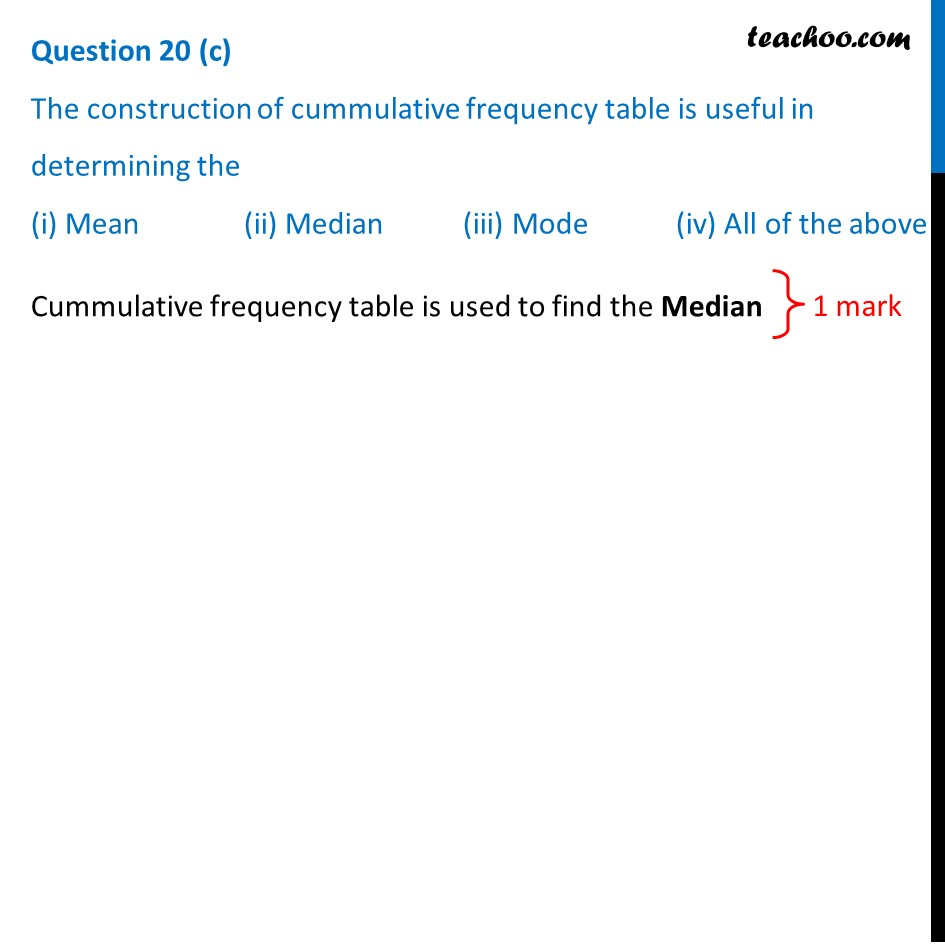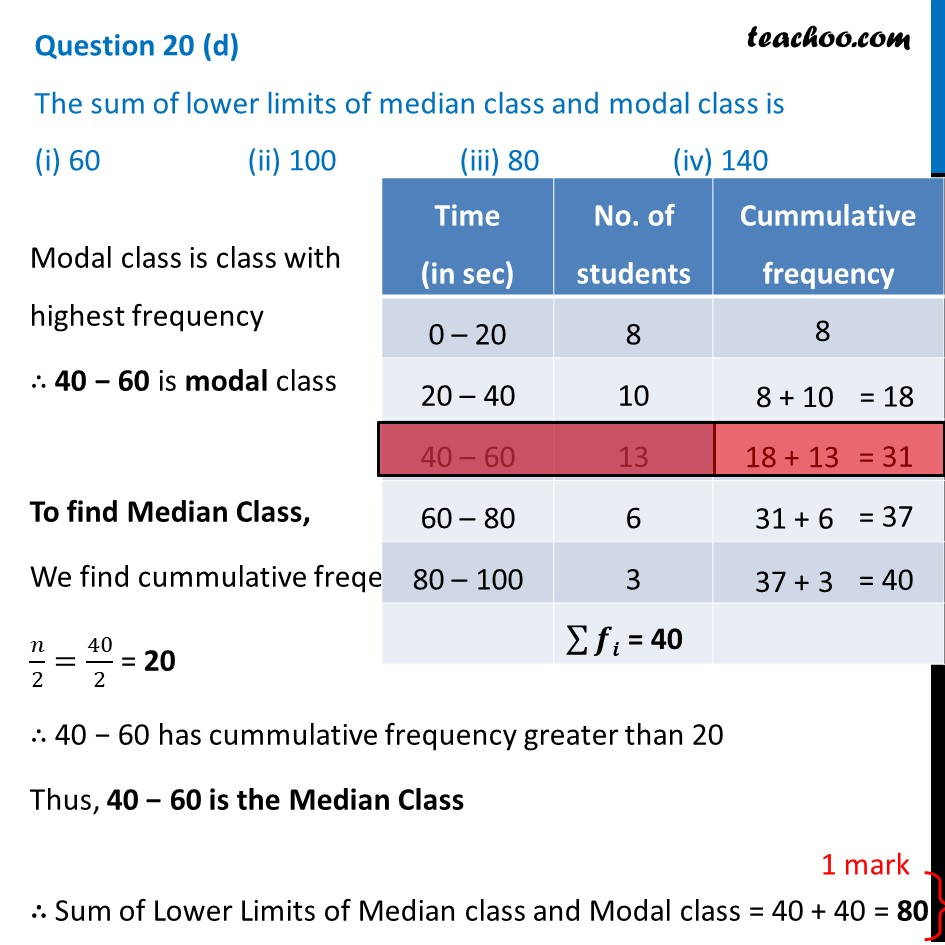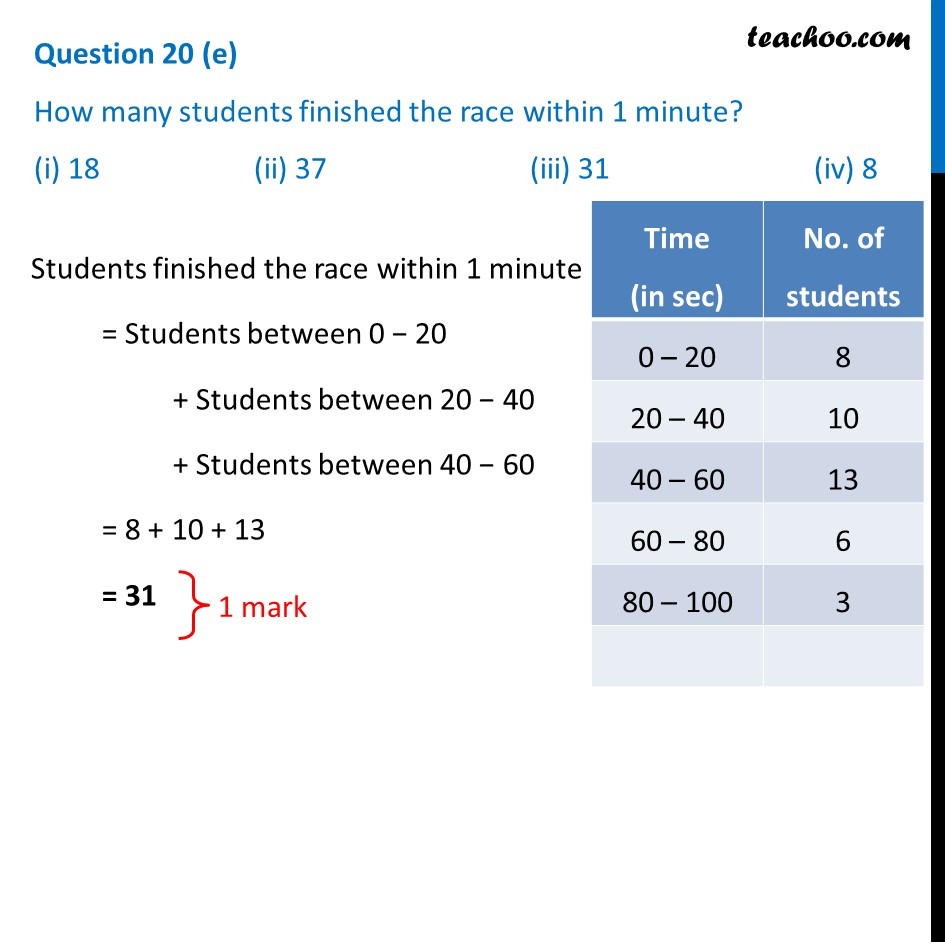1. Class 10
2. Solutions of Sample Papers for Class 10 Boards
3. CBSE Class 10 Sample Paper for 2021 Boards - Maths Standard

Transcript

Question 20 Case Study Based- 4 100m RACE A stopwatch was used to find the time that it took a group of students to run 100 m. (a) Estimate the mean time taken by a student to finish the race. (i) 54 (ii) 63 (iii) 43 (iv) 50 Mean(𝑥 ̅) = (∑▒𝒇𝒊𝒙𝒊)/(∑▒𝒇𝒊) = 1720/40 = 43 Mean time is 43 (b) What wiil be the upper limit of the modal class ? (i) 20 (ii) 40 (iii) 60 (iv) 80 Modal class is class with highest frequency ∴ 40 − 60 is the modal class And Upper limit = 60 (c) The construction of cummulative frequency table is useful in determining the (i) Mean (ii) Median (iii) Mode (iv) All of the aboveCummulative frequency table is used to find the Median (d) The sum of lower limits of median class and modal class is (i) 60 (ii) 100 (iii) 80 (iv) 140 Modal class is class with highest frequency ∴ 40 − 60 is modal class To find Median Class, We find cummulative freqeuncy 𝑛/2=40/2 = 20 ∴ 40 − 60 has cummulative frequency greater than 20 Thus, 40 − 60 is the Median Class ∴ Sum of Lower Limits of Median class and Modal class = 40 + 40 = 80 (e) How many students finished the race within 1 minute? (i) 18 (ii) 37 (iii) 31 (iv) 8 Students finished the race within 1 minute = Students between 0 − 20 + Students between 20 − 40 + Students between 40 − 60 = 8 + 10 + 13 = 31

CBSE Class 10 Sample Paper for 2021 Boards - Maths Standard

Class 10
Solutions of Sample Papers for Class 10 Boards